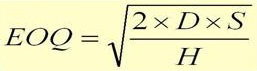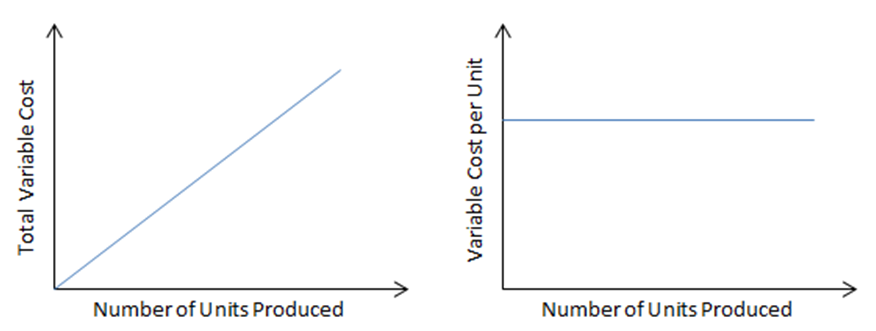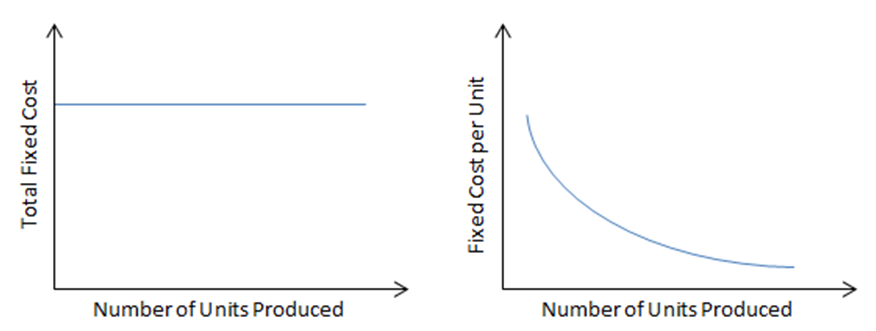Cost Accounting

Cost accounting is the reporting and analysis of a company's cost structure. It is the process of recording and classifying costs incurred in a business in a way that it can be used to improve its management. The main objectives of cost accounting are:

• To calculate cost per unit,
• To determine selling price,
• To record and present cost data to management
• To control and reduce cost
• To calculate profit at each level of activity
• To help management in decision making
• To determine break-even point.

Classification of costs

Cost is classified normally in terms of a managerial objective. Costs are classified either by its function or by its behaviour. Costs can be classified according to the major functions for which the elements are used. For example

• Production/Manufacturing
• Selling and Distribution
• Finance cost

Cost behaviour is the way in which costs are affected by changes in the volume of activity. For example

• variable costs
• fixed costs.
• semi variable / semi fixed costs
• step costs

Direct and Indirect cost

A direct cost is a cost that can be identified in full to a product. For example direct materials, direct labour, direct expenses and variable overheads. Direct costs are variable costs since they vary in proportion to number of units produced.

Indirect costs are incurred in production of goods but which cannot be identified in full to the product. Indirect costs are also known as overhead. Examples of indirect costs are indirect wages, indirect materials and indirect expenses. Indirect cost may be fixed or variable costs. Fixed costs are those costs which do not vary with the level of output.

Direct materials - Direct materials are costs that are directly used in the production of finished goods.

Example

The following information is available for the production of 1 unit

Kg of materials                       3Kg

Price per Kg                            \$4.50/Kg

The cost of materials used to produce 2000 units will be:

= 2 000 units * 3Kg / unit * \$ 4.50/ Kg

= \$ 27 000

In a manufacturing concern there is a separate purchase/procurement department under the control of purchasing officer. The objective is to purchase materials in time so as not to disrupt production. The procedures are as follows: Purchase requisition, Purchase order and delivery of materials. Large manufacturing companies keep inventories of materials. The main purposes for holding inventories are

• to receive discount from suppliers for bulk purchase
• to allow smooth running of production processes
• to meet future shortages

The cost of inventory consist of the purchase price, holding cost, ordering cost and any cost incurred in bringing the inventory to its present location and condition. Economic order quantity (EOQ) is the ideal quantity a company should purchase to minimize inventory costs.D = annual demand

S = cost per order

C = cost per unit

I = percentage holding cost

H = holding cost (s) = I * C

Just in time Inventory (JIT)

JIT is a form of inventory management that requires working closely with suppliers so that raw materials arrive as production is scheduled to begin, but no sooner. The goal is to have the minimum amount of inventory on hand to meet demand. JIT inventory ensures there is enough stock to produce only what you need, when you need it. The goal is to achieve high volume production with minimal inventory on hand and eliminate waste.

Direct Labour

Direct labour refers to the salaries and wages paid to workers that can be directly attributed to the production of specific products. Workers may be paid on a time rate or piece rate basis.

Time rate - Under this method workers are paid on an hourly rate basis.

Piece rate –Direct labour is calculated on the basis of number of units produced by each worker

Example 1

The following information is available to produce one unit

Hours per unit             3 hrs

Rate per hour              \$15

The direct labour cost of producing 1 000 units

= 1000 units * 3 hrs / unit * \$15 / hr

= \$ 45 000

Example 2

A company working at 75% capacity is actually producing 1500 units. Workers are paid at \$ 12 per hour and it takes 2 hours to produce 1 unit. The company received an order of 800 units. Workers are paid at time and a half for the extra units produced during overtime.

75%  = = > 1500units

100% = = > 2000units

Total production = 1500 units + 800 units = 2300 units

 Units Hours Rate Overtime Total 2000 2hrs \$12 - = \$48 000 300 2hrs \$12 1.5 = \$10 800 Total Direct Labour = \$ 58 800

Variable cost

These costs vary directly with the level of output. If number of units produced increases variable cost will increase. Examples of Variable costs:

• Direct materials
• Direct LabourNote : Variable cost per unit always remain the same.

Variable cost                          = No of units produced × Variable cost per unit

Total variable cost                = DM + DL + VO

Total variable cost per unit = DM per unit + DL per unit + VO per unit

Variable cost per unit           = Change in Total cost ÷ Change in Output

Fixed Cost

These costs do not vary with the level of output. Whatever be the level of production, fixed cost always remain the same.Total cost = Variable cost + Fixed cost

Profit = Revenue – Total cost (VC + FC)

For decision making purposes some cost can also be classified as follows:

• Opportunity cost – next best alternative foregone
• Sunk cost – cost that has already been incurred and cannot be recovered
• Step cost – cost that does not change steadily with changes in activity, but rather at discrete points.

Main types of costing

• Marginal costing
• Absorption costing
• Job and Batch costing
• Standard costing
• Activity based costing